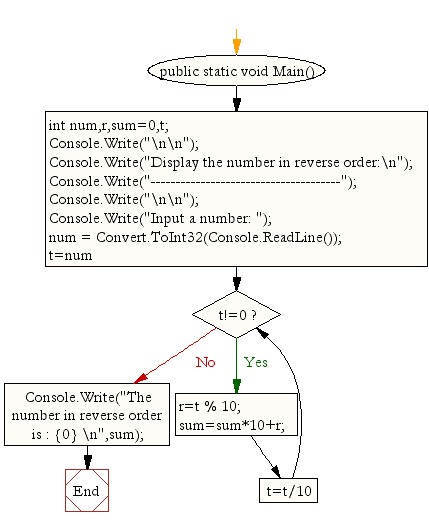﻿ C# - Display the number in reverse order - w3resource# C# Sharp Exercises: Display the number in reverse order

## C# Sharp For Loop: Exercise-37 with Solution

Write a program in C# Sharp to display the number in reverse order.

Pictorial Presentation:Sample Solution:

C# Sharp Code:

``````using System;
public class Exercise37
{
public static void Main()
{
int num,r,sum=0,t;

Console.Write("\n\n");
Console.Write("Display the number in reverse order:\n");
Console.Write("--------------------------------------");
Console.Write("\n\n");

Console.Write("Input a number: ");

for(t=num;t!=0;t=t/10)
{
r=t % 10;
sum=sum*10+r;
}
Console.Write("The number in reverse order is : {0} \n",sum);
}
}
```
```

Sample Output:

```Display the number in reverse order:
--------------------------------------
Input a number: 25
The number in reverse order is : 52
```

Flowchart:C# Sharp Code Editor:

Contribute your code and comments through Disqus.

What is the difficulty level of this exercise?

Test your Programming skills with w3resource's quiz.

﻿